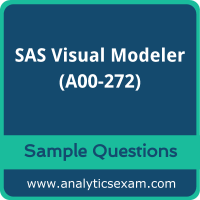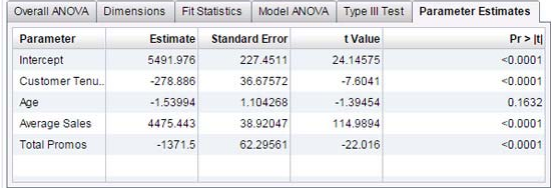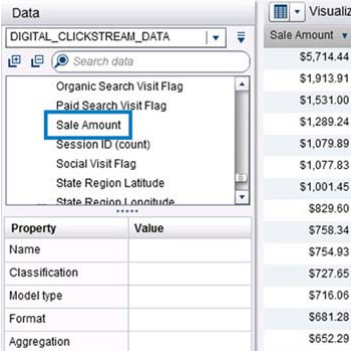# SAS Visual Modeler (A00-272) Certification Exam Sample QuestionsHere are the sample questions which will help you be familiar with SAS Certified Visual Modeler Using SAS Visual Statistics 7.4 (A00-272) exam style and structure. We encourage you to try our Demo SAS Visual Modeler Certification Practice Exam to measure your understanding of exam structure in an environment which simulates the SAS Interactive Model Building and Exploration Using SAS Visual Statistics 7.4 Certification test environment.

To make your preparation more easy for SAS Interactive Model Building and Exploration Using SAS Visual Statistics 7.4 (A00-272) exam, we strongly recommend you to use our Premium SAS Visual Modeler Certification Practice Exam. According to our survey with certified candidates, you can easily score 85% in your actual SAS Certification exam if you can score 100% in our premium SAS Visual Modeler Certification practice exams.

## SAS A00-272 Sample Questions:

01. Which model does not produce score code?
a) Decision Tree using interactive mode
b) Regression using interaction effects
c) Regression using the group by option
d) Decision Tree using the rapid growth option

02. Refer to the exhibit from a linear regression model in SAS Visual Statistics:Based on the table above and assuming a significance level of 0.05, what can be concluded about the linear regression model?
a) The Intercept is an important predictor of the response.
b) Age is a significant predictor of the response.
c) For one one-unit increase in Average Sales, there is an expected increase in the response of 4475.443.
d) For a 1371.5 unit decrease in Total Promos, there is an expected one-unit increase in the response.

03. You have a regression model effect that represents the total amount of sales. In addition to that, you would like to create a model effect that represents the average amount of sales.
Which option should you use?
a) Create an aggregated measure using the Avg aggregation on total amount of sales.
b) Create a calculated item that divides total amount of sales by the total amount of items sold.
c) Create a calculated item by duplicating the original model effect and changing its default aggregation to Average.
d) Create an aggregated measure using the Sum aggregation of total amount of sales divided by the Sum aggregation of total amount of items sold.

04. Your company has a dataset loaded into LASR that represents global sales.
You are a part of a team of analysts that each have responsibility for a certain region of the world.
You decide to create a data source filter to suppress every region but yours. What effect will this have on any new explorations that your teammates create?
a) It will delete all observations that do not match your region.
b) It will have no effect on any observations in the dataset.
c) It will suppress all observations that do not match your region.
d) It will suppress all observations that do not match their corresponding region.

05. Refer to the exhibit:Which is the modeling approach that should be used when fitting the Target Gift Amount variable?
a) Linear regression model with Interaction effects.
b) Generalized linear model with a Poisson distribution and Identity link.
c) Logistic regression model.
d) Generalized linear model with a Normal distribution and Log Link.

06. You perform a logistic regression on a multinomial response variable in SAS Visual Statistics that has 3 levels: Small, Medium, Large. "Large" is specified as the event.
Which statement is true?
a) The other levels are grouped into one non-event.
b) An ordinal logistic regression is performed.
c) A multinomial logistic regression is performed.
d) The other levels are offset to account for exposure.

07. Refer to the exhibit:Which option was not specified in creating the linear regression model using SAS Visual Statistics?
a) interaction term
b) group-by variable
c) variable selection
d) continuous effects

08. You would like to compare multiple models that you've built in SAS Visual Statistics. Which parameters must be the same for all models being compared?
(choose 3)
a) Data Source
b) Assessment Bins
c) Model Type
d) Event Level
e) Response Variable
f) Link Function

09. Refer to the exhibit:Prior to performing a decision tree analysis, you need to assess the default Values of Classification and Model Type Properties for Sale Amount.
The variable represents product purchase amounts from an organization's e-commerce website.
How do you want the decision tree algorithm to treat this variable?
a) Classification = Category & Model Type = Continuous
b) Classification = Measure & Model Type = Discrete
c) Classification = Category & Model Type = Discrete
d) Classification = Measure & Model Type = Continuous

10. Which equation does NOT represent a linear model?
Note: bi are parameters and Xi are variables.
a) y = b0 + b1X1 + b2X2
b) y = b0 + b1X1 + b2X2 + b3(X1X2)
c) y = b0 + b1X1 + (b2/b1)X2
d) y = b0 + b1X1 + b2X1 3

## Answers:

 Question: 1 Answer: a Question: 2 Answer: c Question: 3 Answer: c Question: 4 Answer: b Question: 5 Answer: d Question: 6 Answer: a Question: 7 Answer: b Question: 8 Answer: a, d, e Question: 9 Answer: d Question: 10 Answer: c

Note: Please write us on feedback@analyticsexam.com if you find any data entry error in these SAS Interactive Model Building and Exploration Using SAS Visual Statistics 7.4 (A00-272) sample questions.